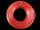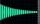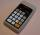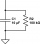# Decibel

By what percentage does the sound intensity increase if the sound intensity level increases by 1 dB?

Correct result:

p =  25.8925 %

#### Solution:We would be pleased if you find an error in the word problem, spelling mistakes, or inaccuracies and send it to us. Thank you!Tips to related online calculators

## Next similar math problems:

• What isWhat is the annual percentage increase in the city when the population has tripled in 20 years?
• Exponential equationFind x, if 625 ^ x = 5 The equation is exponential because the unknown is in the exponential power of 625
• Exponential warmSuppose that a body with temperature T1 is placed in surroundings with temperature T0 different from that of T1. The body will either cool or warm to temperature T(t) after time t, in minutes, where T(t)=T0 + (T1-T0)e^(-kt) . If jello salad with 30 degree
• Electrics - conductorThe wire is 106 meters long at 0 °C and at every temperature increase of 1 °C the length increases by 0.15 mm per 1 m length of wire. Determine a function which represents the overall length of the wire as a function of temperature. What is the length of
• Volume of woodEvery year, at the same time, an increase in the volume of wood in the forest is measured. The increase is regularly p% compared to the previous year. If in 10 years the volume of wood has increased by 10%, what is the number p?
• DemographicsThe population grew in the city in 10 years from 42000 to 54500. What is the average annual percentage increase of population?
• Profit growthThe profit of a company increased by 25% during the year 1992, increased by 40% during the year 1993, decreased by 20% in the year 1994 and increased by 10% during the year 1995. Find the average growth in the profit level over the four years periods?
• Geometric progressionIn geometric progression, a1 = 7, q = 5. Find the condition for n to sum first n members is: sn≤217.
• DecibelA circuit has an input power of 58 mW. Its output power is 6 mW. What is the loss in decibels?
• Semiannually compound interestIf you deposit $5000 into an account paying 8.25% annual interest compounded semiannually, how long until there is$9350 in the account?
• Cube edgesIf the edge length of the cube increases by 50%, how does the volume of this cube increase?
• Growth of woodThe annual growth of wood in the forest is estimated at 2%. In how many years will make the forest volume double?
• ExpIf ?, then n is:
• RC time constantYou introduced 1 Coulomb worth of electrons into the inner volume of a dielectric material with ϵr=6. 30 minutes later, you found that only 36.79% of the electrons were in the inner volume. Determine the conductivity σ of the dielectric material.
• Exponential equationSolve for x: (4^x):0,5=2/64.
• Car valueThe car loses value 15% every year. Determine a time (in years) when the price will be halved.
• CombinationsIf the number of elements increase by 3, it increases the number of combinations of the second class of these elements 5 times. How many are the elements?